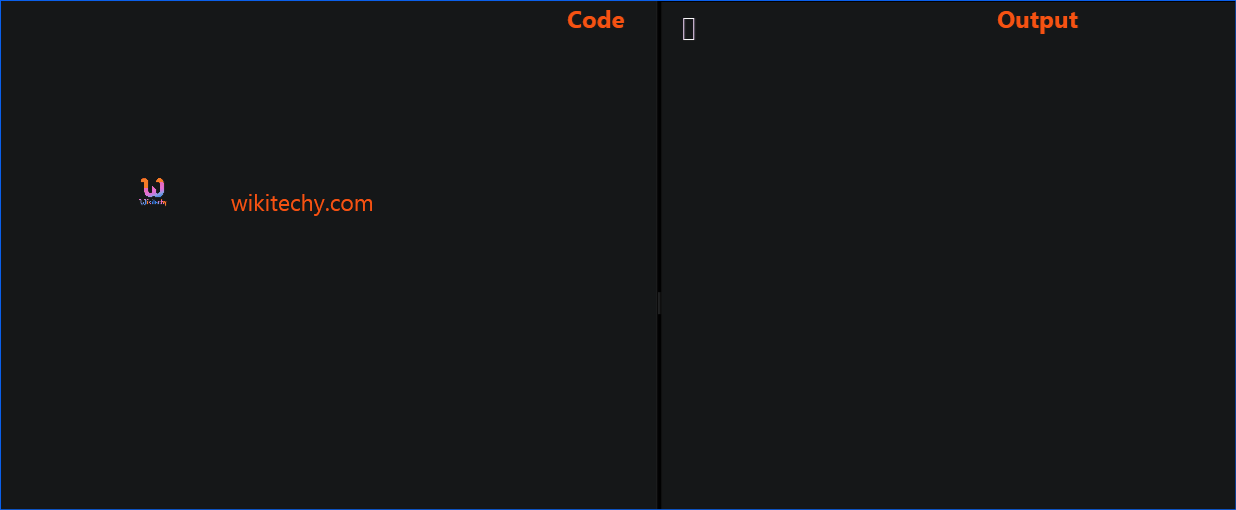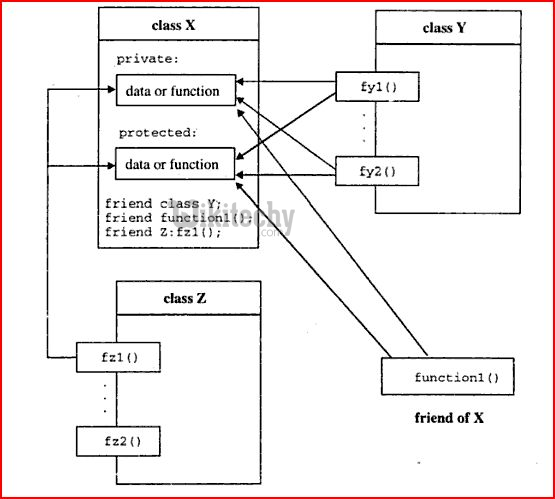# C++ Friend Function | C++ friend Function and friend Classes - Learn C++ - C++ Tutorial - C++ programmingLearn c++ - c++ tutorial - c++-friend-function - c++ examples - c++ programs

## Why We Use Friend Function in C++

• One of the important concepts of OOP is data hiding, i.e., a nonmember function cannot access an object's private or protected data.
• But, sometimes this restriction may force programmer to write long and complex codes. So, there is mechanism built in C++ programming to access private or protected data from non-member functions.
• This is done using a friend function or/and a friend class

## Friend Function in C++

• If a function is defined as a friend function then, the private and protected data of a class can be accessed using the function.
• The complier knows a given function is a friend function by the use of the keyword friend.
•learn c++ tutorials - friend function in c++ Example

• For accessing the data, the declaration of a friend function should be made inside the body of the class (can be anywhere inside class either in private or public section) starting with keyword friend.

## Declaration of friend function in C++

• Now, you can define the friend function as a normal function to access the data of the class. No friend keyword is used in the definition.

## Output

• Here, friend function addFive() is declared inside Distance class. So, the private datameter can be accessed from this function.
• Though this example gives you an idea about the concept of a friend function, it doesn't give show you any meaningful use.
• A more meaningful use would to when you need to operate on objects of two different classes. That's when the friend function can be very helpful.
• You can definitely operate on two objects of different classes without using the friend function but the program will be long, complex and hard to understand.

## Output

• In this program, classes A and B have declared add() as a friend function. Thus, this function can access private data of both class.
• Here, add() function adds the private data numA and numB of two objects objectA andobjectB, and returns it to the main function.
• To make this program work properly, a forward declaration of a class class B should be made as shown in the above example.
• This is because class B is referenced within the class A using code: friend int add(A , B);.

## Friend Class in C++ Programming

• Similarly, like a friend function, a class can also be made a friend of another class using keyword friend. For example:
• When a class is made a friend class, all the member functions of that class becomes friend functions.
• In this program, all member functions of class B will be friend functions of class A.
• Thus, any member function of class B can access the private and protected data of class A.
• But, member functions of class A cannot access the data of class B.
• Remember, friend relation in C++ is only granted, not taken.